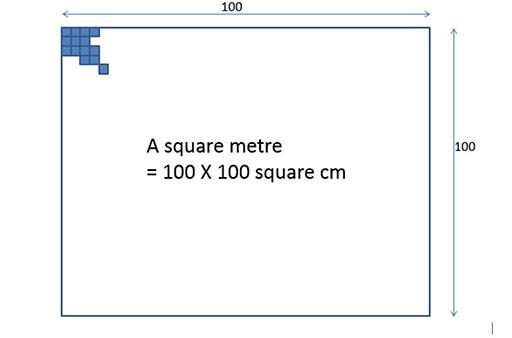Teaching mathematics

Start this free course now. Just create an account and sign in. Enrol and complete the course for a free statement of participation or digital badge if available.

Free course

# 4.2 Converting square metres into square centimetres

If you imagine a tile which is one square metre, then 100 square centimetre tiles would line up along its edge.

You could fit 100 rows of these hundred square centimetre tiles inside the metre square.

That’s 100 × 100 = 10 000 square centimetres in one square metre (Figure 13).Figure 13 A square metre
TM_1